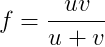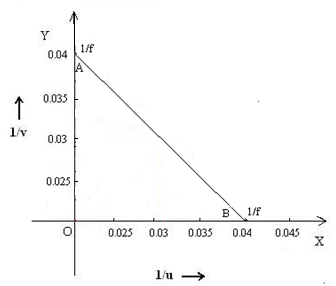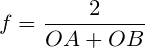The Concave mirror - u-v method

# Materials Required

• Concave mirror
• Stand
• Screen
• Illuminated wire gauze.
• Metre scale

# Real lab Procedure

## By distant object method :

• Fix the given concave mirror on the stand and place it on a table, facing towards a distant object.
• Arrange the screen on the table so that the image of the distant object is obtained on it.
• Measure the distance between mirror and screen using a metre scale. It can be taken as the focal length (f) of the mirror.

## By u-v method :

• Using the focal length obtained by distant object method set the values of u (distance between mirror and object) ranging from 1.5f to 2.5f. Divide the range into a number of equal steps.
• Place the mirror in front of an illuminated wire gauze. It acts as the object.
• Now, fix the mirror at the distance u (which is obtained as 1.5f) from the wire gauze.
• Place the screen on the table facing the mirror in such a way that the reflected image lies on the screen.
• We can adjust the position of the screen to get the clear image of the wire gauze.
• Keeping the distance between object and mirror fixed, adjust the position of screen in order to get the clear image of the object.
• Measure the distance between mirror and wire gauze, as well as mirror and screen. Take these values as u and v respectively.
• Record the values of u and v in a tabular column.
• Calculate the focal length of the given concave mirror by using the relation, f = uv/(u+v).
• Repeat the experiment for different values of u (up to 2.5f) and in each time, measure v and record it in the tabular column.
• Calculate the focal length (f) of the concave mirror each time.
• Calculate the mean of all focal lengths to get the correct focal length of the given concave mirror.
• The focal length of the mirror can also be measured graphically by plotting graphs between u & v, and 1/u & 1/v.
• We can repeat the experiment with concave mirrors of different focal lengths.

# Simulator Procedure (as performed through the Online Labs)

• Select the focal length using the slider.
• Click on the ‘Light On/Off’ button to turn On/Off the wire gauze.
• Select the distance between the mirror and the object (u) using the slider.
• Change the distance between the mirror and the screen (v) suing the slider to get a clear image of the wire gauze on the screen.
• You can calculate the focal length of the mirror using the equation,• You can verify your result by clicking on the ‘Show result’ button.
• To redo the experiment, click the ‘Reset’ button.

# Observations

 No. Distance between the mirror and 1/u (cm-1) 1/v (cm-1)(cm) Object, u (cm) Image, v (cm) 1 2 3 4 5

Mean, f = ………. cm

= ………. ×10-2 m

# Calculations:

Calculate the value of focal length (f) each time and find its mean.

Plot a graph with u along X axis and v along Y axis by taking same scale for drawing the X and Y axes. Draw the bisector OA and join OC and OB. Thus, OC=OB= 2f. Calculate the focal length from this.

Plot a graph with 1/u along X axis and 1/v along Y axis by taking same scale for drawing the X and Y axes. The graph is a straight line intercepting the axes at A and B. Then OA=OB= 1/f. Calculate the focal length from this.u -v Graph                                                          1/u - 1/v Graph

From u-v graph,

OB =….....….cm

OC = ...……..cm=  ........... cm

From 1/u – 1/v graph,

OA =…………cm

OB =…...…... cm= ............ cm

# Results

The focal length of the given concave mirror, f

By u-v method          = ……..×10-2 m

From u-v graph         = .……..×10-2 m

From 1/u- 1/v graph   = ……...×10-2 m

Cite this Simulator: